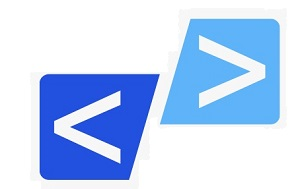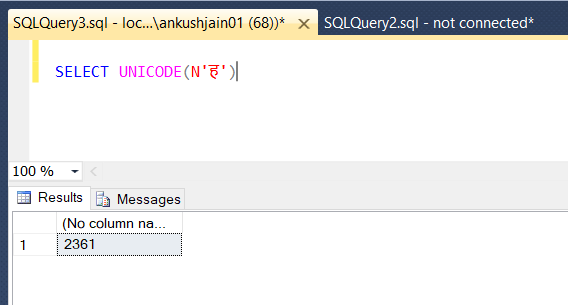﻿ CoderJony - How to check code point value of a Unicode character in SQL Server?CoderJony

# How to check code point value of a Unicode character in SQL Server?

To determine the code-point value of a Unicode character in SQL Server, you can use `UNICODE()` function which is provided by default in SQL Server.

### What is Code Point?

• Every character in a character set (ASCII, UNICODE etc.) is assigned a numeric value, which is known as Code Point.
• A Unicode Code Point is a unique number assigned to each Unicode character.

For example, if you want to determine the code-point value of the English letter `A`, then you simply need to write the below command in SQL Server.

``````SELECT UNICODE('A')
``````

This will output its code-point value.

You may have to prefix `N` before the character if the character is a Non-ASCII character. `N` stands for National Language Character Set.

``````SELECT UNICODE(N'ह')
``````

See the below example image: